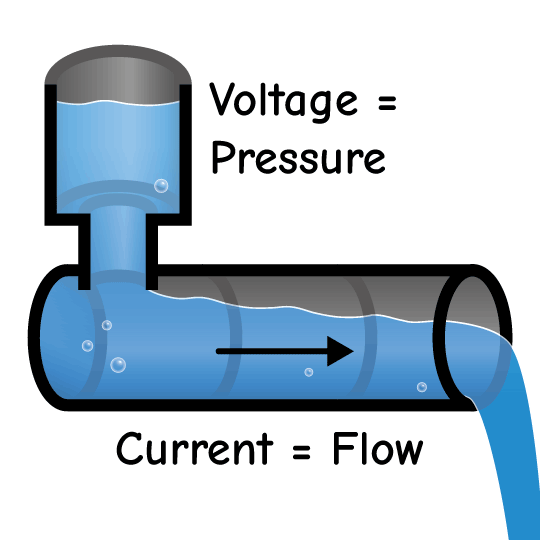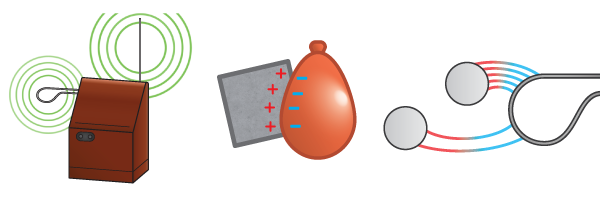# Electricity as Water

When engineers talk about electricity, they are actually talking about two things: voltage and current. To understand the difference, let’s pretend a circuit is made out of pipes holding water. We will replace electrons, the particles that carry electrical charge, with drops of water.Voltage measures how much “water pressure” there is between two points in a circuit. The higher the water level, the more potential energy the water has. This is similar to how a battery gives electrons potential energy. Voltage is measured in Volts (V).

Current measures how much “water flow” there is through an area. The higher the current, the more electric charge moves in a direction. Current is measured in Amps (A) and charge is measured in Coulombs (Q). If a wire has one amp of current, it means one coulomb of charge is flowing through it every second.Voltage and current are related to each other. Just like low water pressure causes water to flow slowly, low voltage will cause a low current. Different electrical parts change how voltage and current interact.

### Click on an Article Below to Keep Learning